## Classification

#### Classification

Direction: In the questions given below there are four figures in each question, out of which one is different from the rest. Identify the odd man out.

1. NA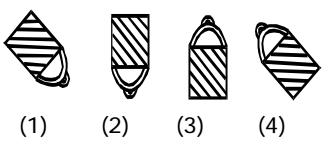1. In the rest of the three, the slanting lines are in the same direction .

##### Correct Option: A

In the rest of the three, the slanting lines are in the same direction, but in option (1) the direction of slanting lines is in the opposite order.

1. NA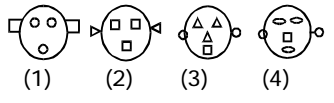1. The eyes and faces of the rest of the three are alike.

##### Correct Option: C

The eyes and faces of the rest of the three are alike. But figure ( 3 ) is different to others .

1. NA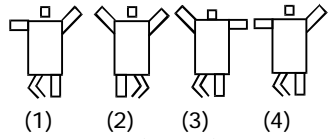1. The hands of the rest of the three are not alike.

##### Correct Option: B

The hands of the rest of the three are not alike. But figure ( 3 ) is different to all three figures .

Direction: From the given responses, find the odd figure out.

1. '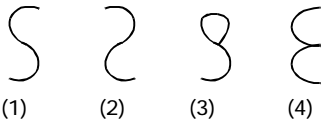1. As we can see that Figure (3) has one closed end.

##### Correct Option: C

As we can see that Figure (3) has one closed end.So , required answer is option C .

Direction: Select the one which is different from the other three responses.

1. NA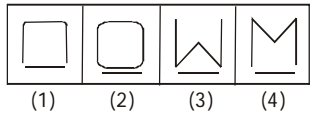1. Except one figure , all other figures have five sides 1.

##### Correct Option: A

Except figure (1), all other figures have five sides 1.